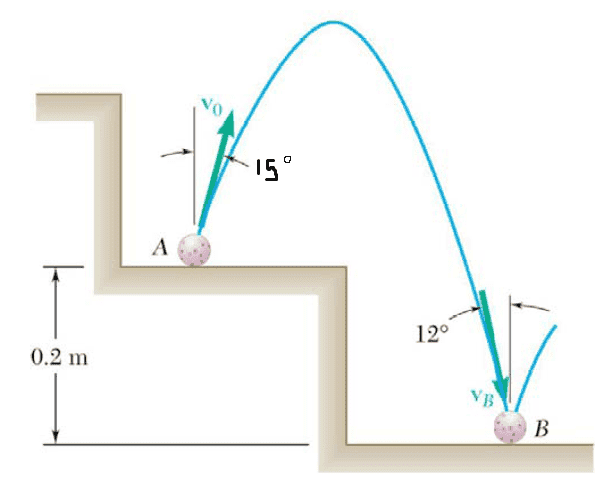# Projectile Motion Ball Drop

## Homework Statement

A Ball is dropped onto a step at point A and rebounds with a velocity Vo at an angle 15 degrees with the vertical. Determine the value of V0 knowing that just before the ball bounces at Point B its velocity VB forms an angle of 12 degrees with the vertical.X = Vt
Y = Vt - 0.5gt2

## The Attempt at a Solution

X = (VoSin15)t
Y = (VoCos15)t -0.5gt2

I am not sure how to make use of the other information provided.

lewando
Homework Helper
Gold Member
The most relevant equation:

Vy(t)^2 = Vy0^2 + 2g (y(t) - y0)

because we need to use the 0.2m y-displacement.

Also noteworthy:
Vx0 = V0 sin(15)
VxT = VT sin(12)
Vx0 = VxT